In this section, we will look at the properties of matrix addition. These properties include the dimension property for addition, commutative property, and associative property. Note that for the dimension property, we are allowed to add or subtract two matrices with the same dimensions. The commutative property states that changing the order of the addition or subtraction of two matrices lead to the same result. For the associative property, changing what matrices you add or subtract one will lead to the same answer. There are also matrix addition properties for identity and zero matrices as well. Adding a zero matrix with another matrix (call it A) will give back A. Multiplying an identity matrix with another matrix (call it B) will give back B.

#### Lessons

Let the matrices $X,Y$ and $Z$ have equal dimensions. Then we have the following matrix addition properties:

If $X$ and $Y$ has the same dimensions, then $X+Y$ also has the same dimensions.
Commutative property
$X+Y=Y+X$
Associative property
$(X+Y)+Z=X+(Y+Z)$

There are also some matrix addition properties with the identity and zero matrix.

Property for the zero matrix
There is always a zero matrix $O$ such that $O+X=X$ for any matrix $X$.
Property for the identity matrix
Let $X$ be a $n$ by $n$ matrix. Then there is an identity matrix $I_n$ such that $I_n \cdot X=X$.
• Introduction

• 1.
You are given that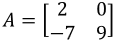and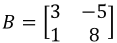and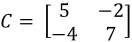. Verify the following properties:
a)
Commutative property $(A+B=B+A)$

b)
Associative property $(A+B)+C=A+(B+C)$

c)

• 2.
You are given that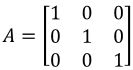and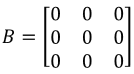and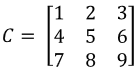. Verify the following properties:
a)
Commutative property $(A+B=B+A)$

b)
Associative property $(A+B)+C=A+(B+C)$

c)
Let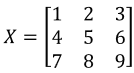. Verify the zero matrix property.
Let $X$ be a 2 x 2 matrix. Show that $I_n \cdot X=X$.